﻿ 低频脉动强化换热实验研究
 舰船科学技术2019, Vol. 41Issue (2): 106-109PDF

Experimental study on heat transfer enhancement of low frequency pulsation
WANG Jun, GE Yi-ru, PAN Chao-feng, CHEN Ning
School of Energy and Power Engineering, Jiangsu University of Science and Technology, Zhenjiang 212003, China
Abstract: In this paper, the low-frequency pulsating flow on the intensified heat transfer is studied in view of the application of pulsating intensified heat transfer technology has a wide application prospect in improving the heat transfer performance and scale removal of ship equipment. The pulsating flow with the electromagnetic valve as the pulsating source is added to the upstream of the cooling water end of the plate heat exchanger. The heat exchange ratio Em was used to characterize the heat transfer effect. Change the Reynolds number, pulsation frequency and pulsation amplitude of the pulsating flow to explore the relationship between the pulse parameters and the heat exchange effect. The experimental results show that the pulsating flow has the effect of enhanced heat transfer. Its enhanced heat transfer capacity is affected by the fluid flow state, pulsating frequency and other factors. In some cases, pulsating flow can lead to weaken heat transfer. In some cases, pulsating flow will lead to heat transfer weakening.
Key words: pulsating     strengthening heat transfer     pulsating frequency     plate heat exchanger
0 引　言

1 脉动强化换热实验 1.1 实验设计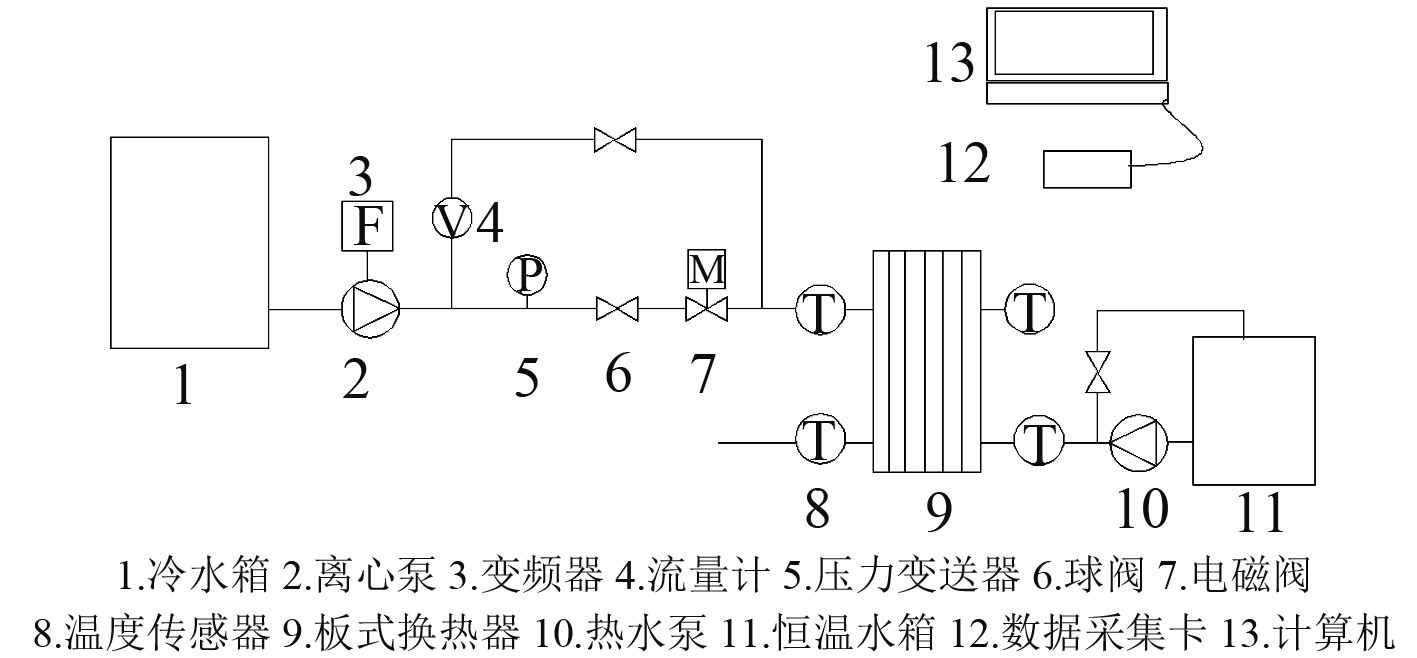图 1 脉动强化换热实验系统原理图 Fig. 1 System schematic diagram of pulsating enhanced heat transfer experiment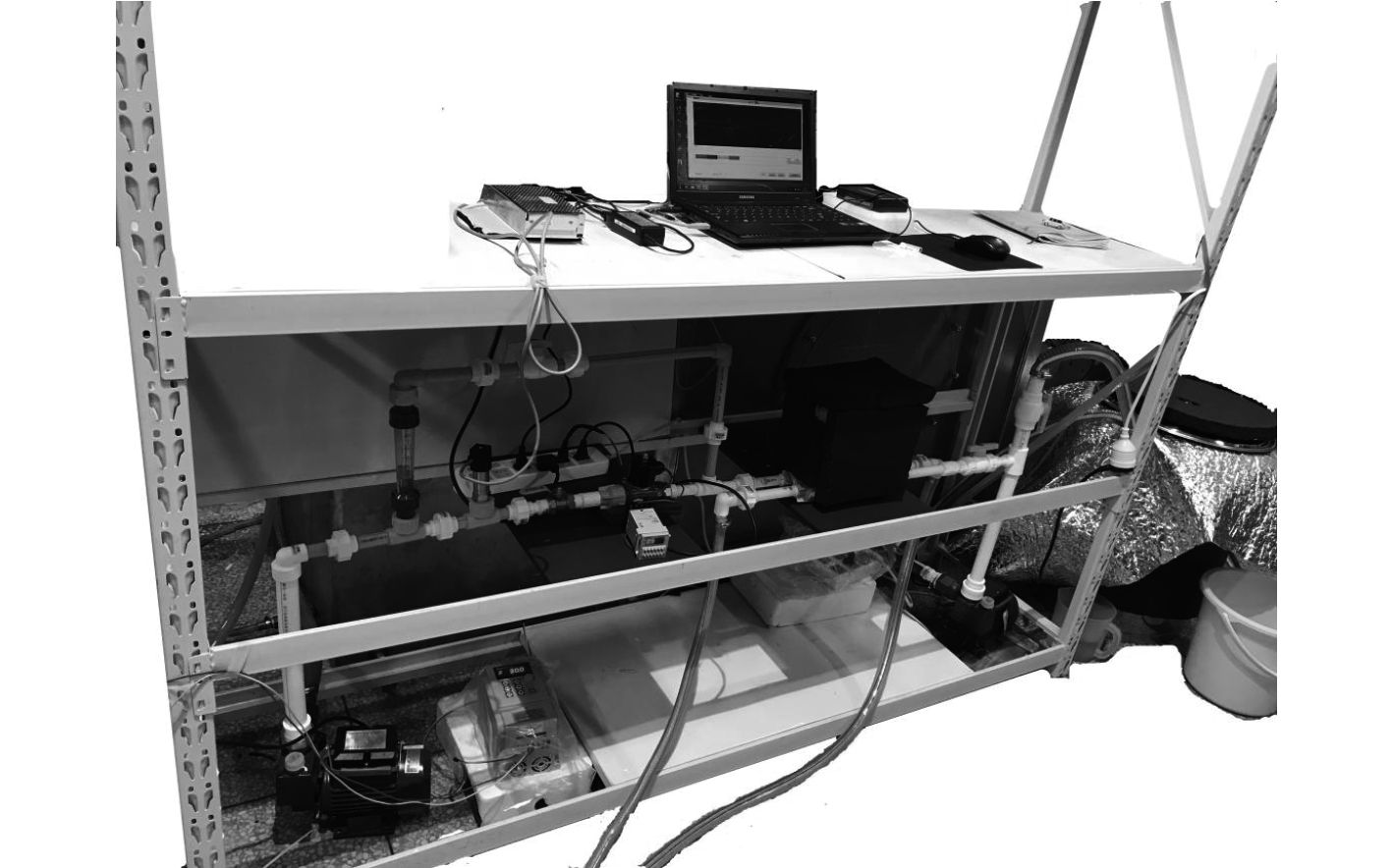图 2 脉动强化换热实验系统实物图 Fig. 2 System physical diagram of pulsating enhanced heat transfer experiment
1.2 实验计算

 ${Q_C} = c \cdot \overline m {}_c \cdot {\overline {\Delta T} _c} = c \cdot \overline {{m_c}} \cdot \left( {\overline {{T_{co}}} - \overline {{T_{cin}}} } \right){\text{。}}$ (1)

 ${Q_h} = c \cdot {\overline m _h} \cdot {\overline {\Delta T} _h} = c \cdot \overline {{m_h}} \cdot \left( {\overline {{T_{hin}}} - \overline {{T_{ho}}} } \right){\text{。}}$ (2)

 $K = \frac{Q}{{A\Delta {t_m}}}{\text{。}}$ (3)
 $Q = \frac{{{Q_c}{Q_h}}}{2}{\text{，}}$ (4)
 $\Delta {t_m} = \frac{{\Delta {t_{\max }} - \Delta {t_{\min }}}}{{\ln \left( {\frac{{\Delta {t_{\max }}}}{{\Delta {t_{\min }}}}} \right)}}{\text{。}}$ (5)

 ${E_{\rm{m}}} = \frac{{{K_p}}}{{{K_s}}}{\text{。}}$ (6)

2 实验结果及数据分析

2.1 强化换热比Em与雷诺数Re的关系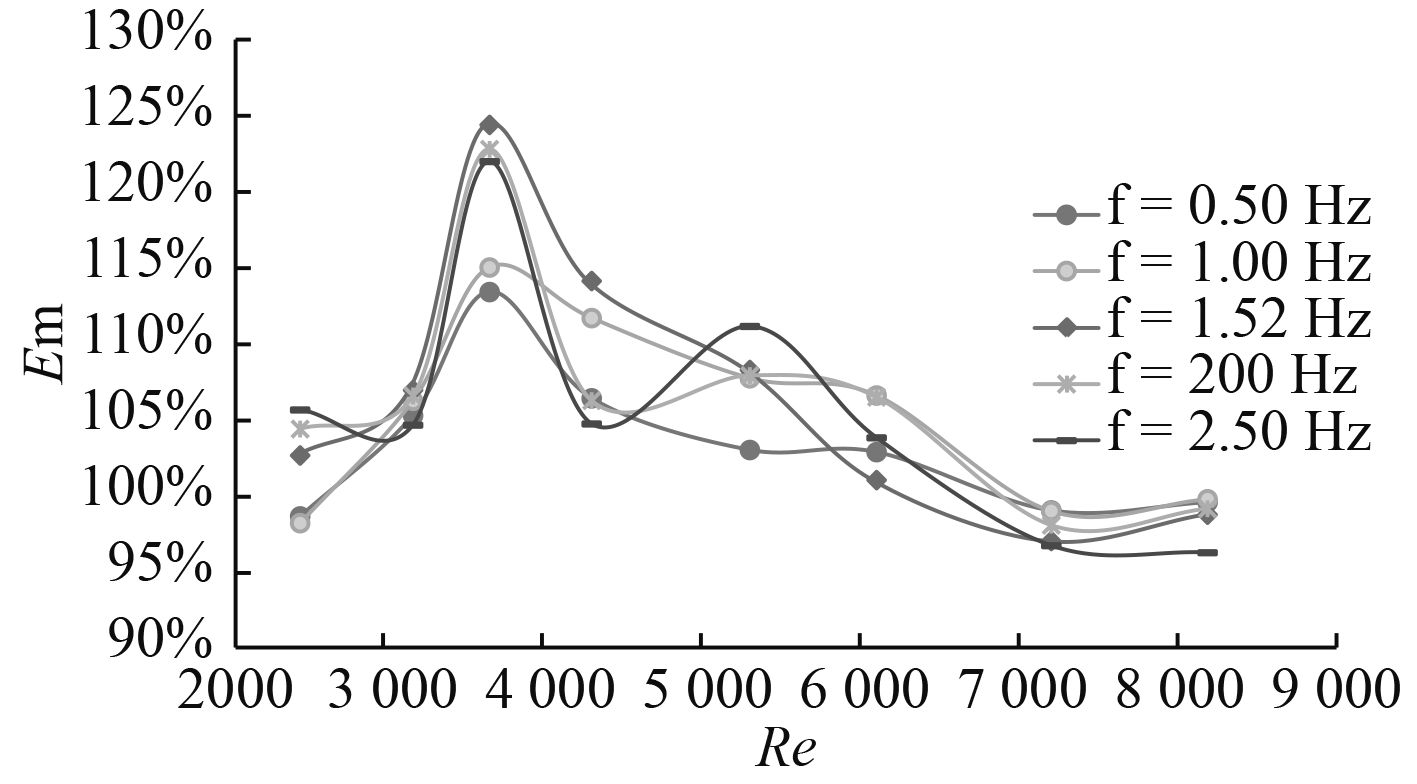图 3 脉动振幅A1下强化换热比Em与雷诺数Re的关系 Fig. 3 The relationship between the reynolds number and enhanced heat exchange ratio under the pulsation amplitude of A1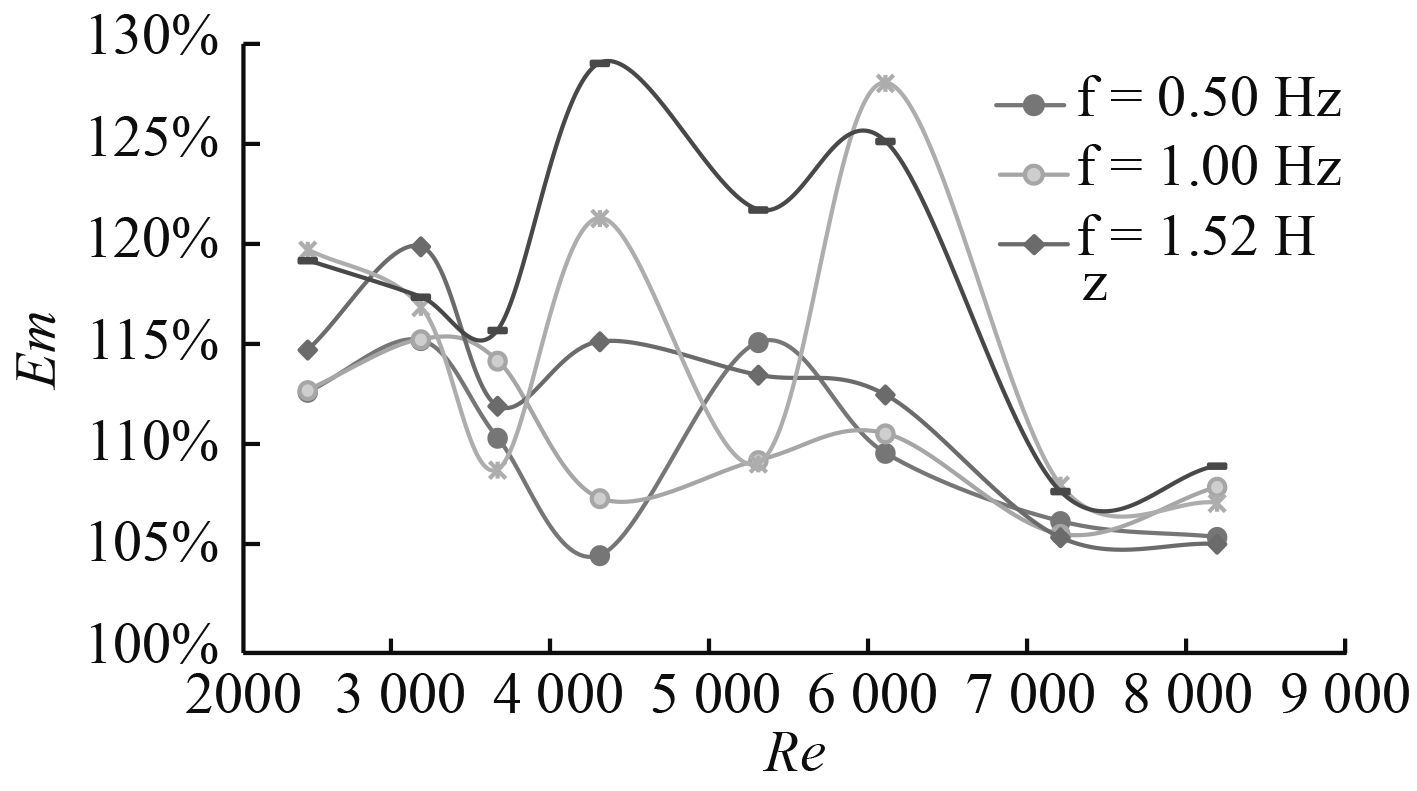图 4 脉动振幅A2下强化换热比与雷诺数的关系 Fig. 4 The relationship between the reynolds number and enhanced heat exchange ratio under the pulsation amplitude of A2

2.2 不同脉动频率下换热结果分析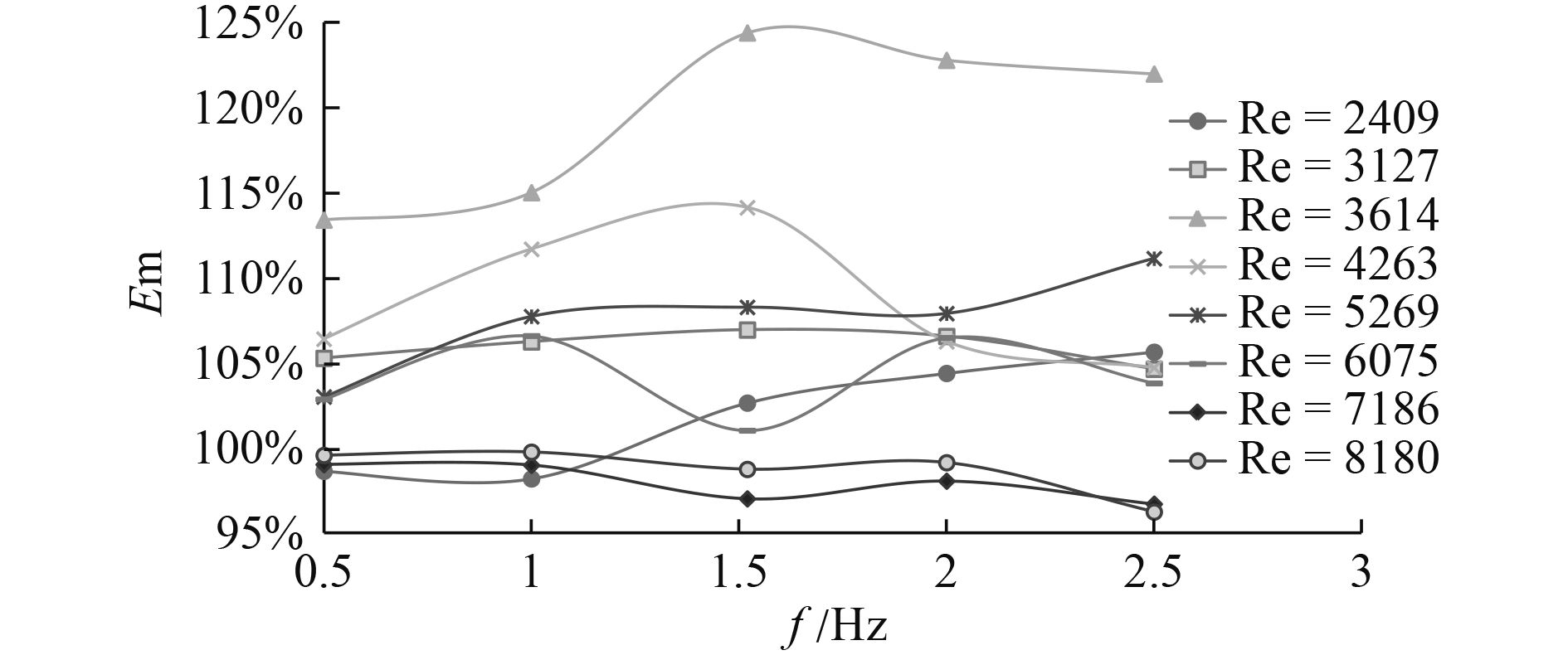图 5 脉动振幅A1下强化换热比Em与脉动频率f的关系 Fig. 5 The relationship between the pulsating frequency and the heat exchange ratio under the pulsation amplitude of A1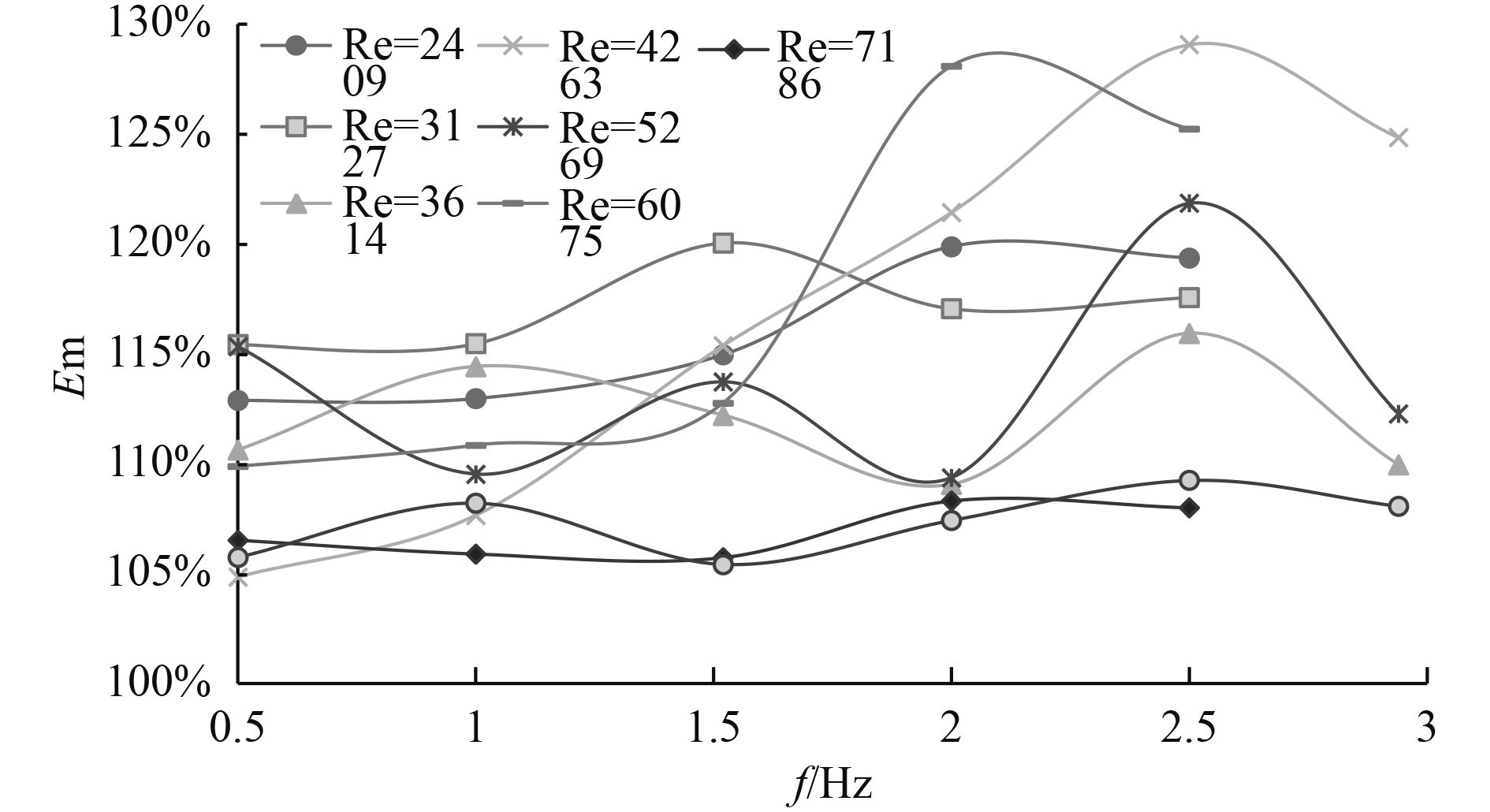图 6 脉动振幅A2下强化换热比Em与脉动频率f的关系 Fig. 6 The relationship between the pulsating frequency and the heat exchange ratio under the pulsation amplitude of A2

2.3 不同脉动振幅下换热结果分析

2.4 脉动振幅A1A2下的最佳脉动频率fo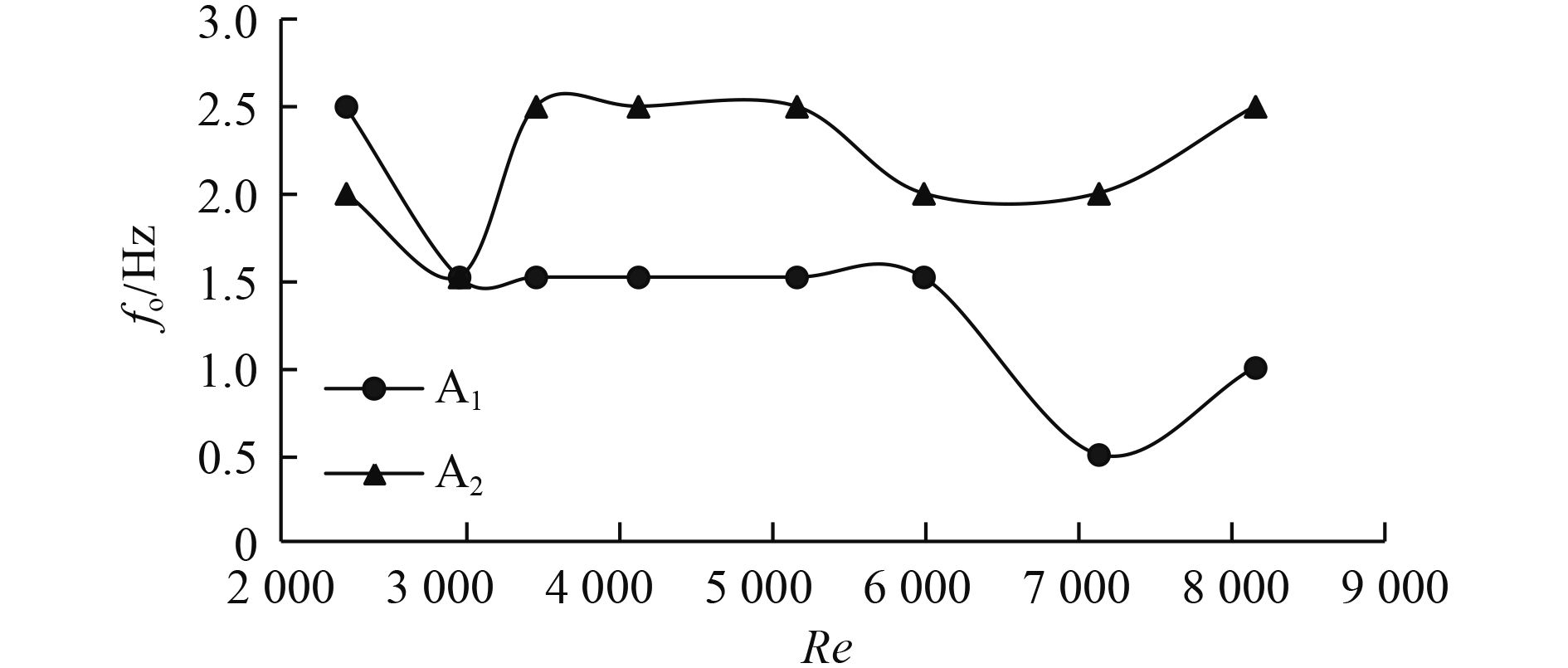图 7 脉动振幅A1，A2下最佳脉动频率fo与雷诺数Re的关系 Fig. 7 The relationship between the optimum pulsating frequency and the Reynolds number under the pulsation amplitude of A1 and A2

3 结　语

1）过渡阶段为最佳强化换热区；在旺盛湍流阶段，可能会出现脉动流弱化换热效果的现象；

2）在层流、过渡、旺盛湍流阶段，脉动频率对脉动流强化换热效果的影响较小；在湍流阶段，脉动频率对换热效果的影响最为强烈；

3）增加脉动振幅对脉动流强化换热能力有一定的提升作用，但在一些情况下，脉动流在较高脉动振幅下的强化换热效果反而降低；

4）在各实验工况下，存在最佳脉动频率fo，其值与流体流动状态、脉动振幅等因素有关；

5）总体而言，在实验工况下，脉动流具有一定的强化换热效果。实验在脉动振幅A2下，Re=4 263，f=2.5 Hz，d=50%时所获得的最佳强化换热比Em达到129.07%。

  HAVEMANN H A, NARAYANRAO N N. Heat transfer inpulsating flow[J]. Nature, 1954, 7(4418): 41.  A. E. ZOHIR. Heat transfer Characteristics in a heat exchanger for turbulent pulsating water flow with different amplitudes[J]. Journal of American Science, 2012, 8(2).  KARAMERCAN, Gainer J. The effect of pulsations on heat transfer[J]. Industrial & Engineering Chemistry Fundamentals, 1979, 18(1): 11-15.  JUBER T. P, MANISH H. A.. Experimental Investigation of Heat Transfer Characteristics of Pulsating Flow in Pipe[J]. International Journal of Current Engineering and Technology, 2016(6): 1515-1522.  JIN D X, LEE Y P, LEE D Y. Effects of the pulsating flow agitation on the heat transfer in a triangular grooved channel[J]. Heat Mass Transfer, 2007(50): 3062-3071. 54, 7(4418): 41.  MOHAMMAD J, MOUSA F.. Pulsating flow effects on convection heat transfer in a corrugated channel:A LBM approach[J]. International Communications in Heat and Mass Transfer 45, 2015, 146-154.  ZOHIR A. E.. The influence of pulsation on heat transter in a heat exchanger for parallel and counter water flows[J]. New York Science Journal, 2011(6): 4-6.  LI Hongxia, HUAI Xiulan. Experimental study on hydraulic cavitation and descaling[J]. Journal of Industrial and Thermal Physics, 2011, 31(9): 1531-1534.